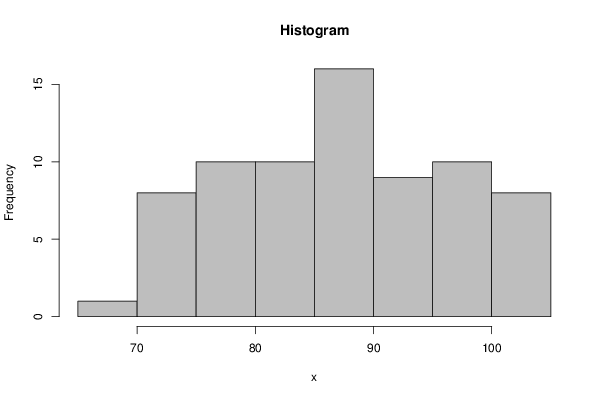## Free Statistics

of Irreproducible Research!

Author's title
Author*Unverified author*
R Software Modulerwasp_histogram.wasp
Title produced by softwareHistogram
Date of computationSat, 29 Dec 2012 09:54:13 -0500
Cite this page as followsStatistical Computations at FreeStatistics.org, Office for Research Development and Education, URL https://freestatistics.org/blog/index.php?v=date/2012/Dec/29/t1356793911kb4j4wvpzwv99rs.htm/, Retrieved Wed, 04 Oct 2023 00:35:08 +0000
Statistical Computations at FreeStatistics.org, Office for Research Development and Education, URL https://freestatistics.org/blog/index.php?pk=204880, Retrieved Wed, 04 Oct 2023 00:35:08 +0000
QR Codes:Original text written by user:
IsPrivate?No (this computation is public)
User-defined keywords
Estimated Impact110
Family? (F = Feedback message, R = changed R code, M = changed R Module, P = changed Parameters, D = changed Data)
-     [Univariate Data Series] [prijs vliegtuigti...] [2012-09-21 20:50:58] [74be16979710d4c4e7c6647856088456]
- RMPD    [Histogram] [gemiddelde prijze...] [2012-12-29 14:54:13] [d0e7cd87186a15776b36563906a5538f] [Current]
- R P       [Histogram] [] [2012-12-29 15:18:06] [770959544eacfa1f4175753a9a1bfc9b]
- RMP       [Kernel Density Estimation] [gemiddelde prijze...] [2012-12-29 15:25:00] [770959544eacfa1f4175753a9a1bfc9b]
- R         [Histogram] [] [2013-01-15 20:44:26] [74be16979710d4c4e7c6647856088456]
- R P       [Histogram] [] [2013-01-15 20:54:04] [770959544eacfa1f4175753a9a1bfc9b]
- R           [Histogram] [] [2013-01-15 21:00:20] [770959544eacfa1f4175753a9a1bfc9b]
Feedback Forum

Post a new message
Dataseries X:
86.86
86.79
82.52
86.87
81.62
82.66
89.87
92.04
79.74
77.75
79.12
76.37
75.01
77.6
77.81
81.7
76.47
74.72
84.43
86.72
70.99
75.43
74.14
73.3
71.97
69.27
74.13
76.4
72.26
72.1
87.82
91.62
82.69
85.76
86.87
93.09
83.73
84.49
87.37
89.13
83.2
83.77
93.68
93.09
88.59
87.88
87.89
89.38
89.13
89.58
90.22
91.44
91.04
92.1
97.54
99.12
100
99.68
100.08
99.9
99.63
99.45
99.63
99.46
96.91
97.65
102.1
103.57
104.59
104.79
101.31
104.8


 Summary of computational transaction Raw Input view raw input (R code) Raw Output view raw output of R engine Computing time 2 seconds R Server 'George Udny Yule' @ yule.wessa.net

\begin{tabular}{lllllllll}
\hline
Summary of computational transaction \tabularnewline
Raw Input & view raw input (R code)  \tabularnewline
Raw Output & view raw output of R engine  \tabularnewline
Computing time & 2 seconds \tabularnewline
R Server & 'George Udny Yule' @ yule.wessa.net \tabularnewline
\hline
\end{tabular}
%Source: https://freestatistics.org/blog/index.php?pk=204880&T=0

[TABLE]
[ROW][C]Summary of computational transaction[/C][/ROW]
[ROW][C]Raw Input[/C][C]view raw input (R code) [/C][/ROW]
[ROW][C]Raw Output[/C][C]view raw output of R engine [/C][/ROW]
[ROW][C]Computing time[/C][C]2 seconds[/C][/ROW]
[ROW][C]R Server[/C][C]'George Udny Yule' @ yule.wessa.net[/C][/ROW]
[/TABLE]
Source: https://freestatistics.org/blog/index.php?pk=204880&T=0

Globally Unique Identifier (entire table): ba.freestatistics.org/blog/index.php?pk=204880&T=0

As an alternative you can also use a QR Code:

The GUIDs for individual cells are displayed in the table below:

 Summary of computational transaction Raw Input view raw input (R code) Raw Output view raw output of R engine Computing time 2 seconds R Server 'George Udny Yule' @ yule.wessa.net

 Frequency Table (Histogram) Bins Midpoint Abs. Frequency Rel. Frequency Cumul. Rel. Freq. Density [65,70[ 67.5 1 0.013889 0.013889 0.002778 [70,75[ 72.5 8 0.111111 0.125 0.022222 [75,80[ 77.5 10 0.138889 0.263889 0.027778 [80,85[ 82.5 10 0.138889 0.402778 0.027778 [85,90[ 87.5 16 0.222222 0.625 0.044444 [90,95[ 92.5 9 0.125 0.75 0.025 [95,100[ 97.5 10 0.138889 0.888889 0.027778 [100,105] 102.5 8 0.111111 1 0.022222

\begin{tabular}{lllllllll}
\hline
Frequency Table (Histogram) \tabularnewline
Bins & Midpoint & Abs. Frequency & Rel. Frequency & Cumul. Rel. Freq. & Density \tabularnewline
[65,70[ & 67.5 & 1 & 0.013889 & 0.013889 & 0.002778 \tabularnewline
[70,75[ & 72.5 & 8 & 0.111111 & 0.125 & 0.022222 \tabularnewline
[75,80[ & 77.5 & 10 & 0.138889 & 0.263889 & 0.027778 \tabularnewline
[80,85[ & 82.5 & 10 & 0.138889 & 0.402778 & 0.027778 \tabularnewline
[85,90[ & 87.5 & 16 & 0.222222 & 0.625 & 0.044444 \tabularnewline
[90,95[ & 92.5 & 9 & 0.125 & 0.75 & 0.025 \tabularnewline
[95,100[ & 97.5 & 10 & 0.138889 & 0.888889 & 0.027778 \tabularnewline
[100,105] & 102.5 & 8 & 0.111111 & 1 & 0.022222 \tabularnewline
\hline
\end{tabular}
%Source: https://freestatistics.org/blog/index.php?pk=204880&T=1

[TABLE]
[ROW][C]Frequency Table (Histogram)[/C][/ROW]
[ROW][C]Bins[/C][C]Midpoint[/C][C]Abs. Frequency[/C][C]Rel. Frequency[/C][C]Cumul. Rel. Freq.[/C][C]Density[/C][/ROW]
[ROW][C][65,70[[/C][C]67.5[/C][C]1[/C][C]0.013889[/C][C]0.013889[/C][C]0.002778[/C][/ROW]
[ROW][C][70,75[[/C][C]72.5[/C][C]8[/C][C]0.111111[/C][C]0.125[/C][C]0.022222[/C][/ROW]
[ROW][C][75,80[[/C][C]77.5[/C][C]10[/C][C]0.138889[/C][C]0.263889[/C][C]0.027778[/C][/ROW]
[ROW][C][80,85[[/C][C]82.5[/C][C]10[/C][C]0.138889[/C][C]0.402778[/C][C]0.027778[/C][/ROW]
[ROW][C][85,90[[/C][C]87.5[/C][C]16[/C][C]0.222222[/C][C]0.625[/C][C]0.044444[/C][/ROW]
[ROW][C][90,95[[/C][C]92.5[/C][C]9[/C][C]0.125[/C][C]0.75[/C][C]0.025[/C][/ROW]
[ROW][C][95,100[[/C][C]97.5[/C][C]10[/C][C]0.138889[/C][C]0.888889[/C][C]0.027778[/C][/ROW]
[ROW][C][100,105][/C][C]102.5[/C][C]8[/C][C]0.111111[/C][C]1[/C][C]0.022222[/C][/ROW]
[/TABLE]
Source: https://freestatistics.org/blog/index.php?pk=204880&T=1

Globally Unique Identifier (entire table): ba.freestatistics.org/blog/index.php?pk=204880&T=1

As an alternative you can also use a QR Code:

The GUIDs for individual cells are displayed in the table below:

 Frequency Table (Histogram) Bins Midpoint Abs. Frequency Rel. Frequency Cumul. Rel. Freq. Density [65,70[ 67.5 1 0.013889 0.013889 0.002778 [70,75[ 72.5 8 0.111111 0.125 0.022222 [75,80[ 77.5 10 0.138889 0.263889 0.027778 [80,85[ 82.5 10 0.138889 0.402778 0.027778 [85,90[ 87.5 16 0.222222 0.625 0.044444 [90,95[ 92.5 9 0.125 0.75 0.025 [95,100[ 97.5 10 0.138889 0.888889 0.027778 [100,105] 102.5 8 0.111111 1 0.022222PNG link Postscript link PDF link

Parameters (Session):
par2 = grey ; par3 = FALSE ; par4 = Unknown ;
Parameters (R input):
par1 = ; par2 = grey ; par3 = FALSE ; par4 = Unknown ;
R code (references can be found in the software module):
par4 <- 'Unknown'par3 <- 'FALSE'par2 <- 'grey'par1 <- '20'par1 <- as.numeric(par1)if (par3 == 'TRUE') par3 <- TRUEif (par3 == 'FALSE') par3 <- FALSEif (par4 == 'Unknown') par1 <- as.numeric(par1)if (par4 == 'Interval/Ratio') par1 <- as.numeric(par1)if (par4 == '3-point Likert') par1 <- c(1:3 - 0.5, 3.5)if (par4 == '4-point Likert') par1 <- c(1:4 - 0.5, 4.5)if (par4 == '5-point Likert') par1 <- c(1:5 - 0.5, 5.5)if (par4 == '6-point Likert') par1 <- c(1:6 - 0.5, 6.5)if (par4 == '7-point Likert') par1 <- c(1:7 - 0.5, 7.5)if (par4 == '8-point Likert') par1 <- c(1:8 - 0.5, 8.5)if (par4 == '9-point Likert') par1 <- c(1:9 - 0.5, 9.5)if (par4 == '10-point Likert') par1 <- c(1:10 - 0.5, 10.5)bitmap(file='test1.png')if(is.numeric(x)) {if (is.na(par1)) {myhist<-hist(x,col=par2,main=main,xlab=xlab,right=par3)} else {if (par1 < 0) par1 <- 3if (par1 > 50) par1 <- 50myhist<-hist(x,breaks=par1,col=par2,main=main,xlab=xlab,right=par3)}} else {plot(mytab <- table(x),col=par2,main='Frequency Plot',xlab=xlab,ylab='Absolute Frequency')}dev.off()if(is.numeric(x)) {myhistn <- length(x)load(file='createtable')a<-table.start()a<-table.row.start(a)a<-table.element(a,hyperlink('histogram.htm','Frequency Table (Histogram)',''),6,TRUE)a<-table.row.end(a)a<-table.row.start(a)a<-table.element(a,'Bins',header=TRUE)a<-table.element(a,'Midpoint',header=TRUE)a<-table.element(a,'Abs. Frequency',header=TRUE)a<-table.element(a,'Rel. Frequency',header=TRUE)a<-table.element(a,'Cumul. Rel. Freq.',header=TRUE)a<-table.element(a,'Density',header=TRUE)a<-table.row.end(a)crf <- 0if (par3 == FALSE) mybracket <- '[' else mybracket <- ']'mynumrows <- (length(myhist$breaks)-1)for (i in 1:mynumrows) {a<-table.row.start(a)if (i == 1)dum <- paste('[',myhist$breaks[i],sep='')elsedum <- paste(mybracket,myhist$breaks[i],sep='')dum <- paste(dum,myhist$breaks[i+1],sep=',')if (i==mynumrows)dum <- paste(dum,']',sep='')elsedum <- paste(dum,mybracket,sep='')a<-table.element(a,dum,header=TRUE)a<-table.element(a,myhist$mids[i])a<-table.element(a,myhist$counts[i])rf <- myhist$counts[i]/ncrf <- crf + rfa<-table.element(a,round(rf,6))a<-table.element(a,round(crf,6))a<-table.element(a,round(myhist$density[i],6))a<-table.row.end(a)}a<-table.end(a)table.save(a,file='mytable.tab')} else {mytabreltab <- mytab / sum(mytab)n <- length(mytab)load(file='createtable')a<-table.start()a<-table.row.start(a)a<-table.element(a,'Frequency Table (Categorical Data)',3,TRUE)a<-table.row.end(a)a<-table.row.start(a)a<-table.element(a,'Category',header=TRUE)a<-table.element(a,'Abs. Frequency',header=TRUE)a<-table.element(a,'Rel. Frequency',header=TRUE)a<-table.row.end(a)for (i in 1:n) {a<-table.row.start(a)a<-table.element(a,labels(mytab)\$x[i],header=TRUE)a<-table.element(a,mytab[i])a<-table.element(a,round(reltab[i],4))a<-table.row.end(a)}a<-table.end(a)table.save(a,file='mytable1.tab')}